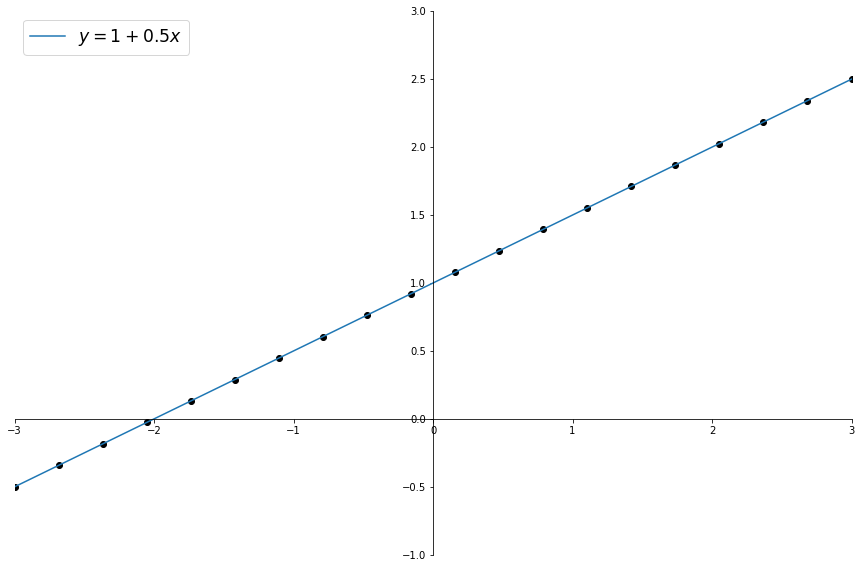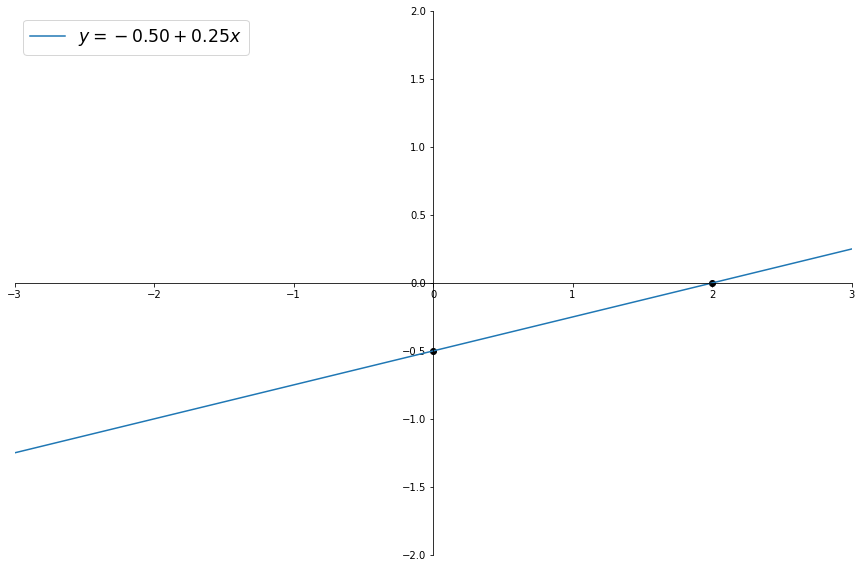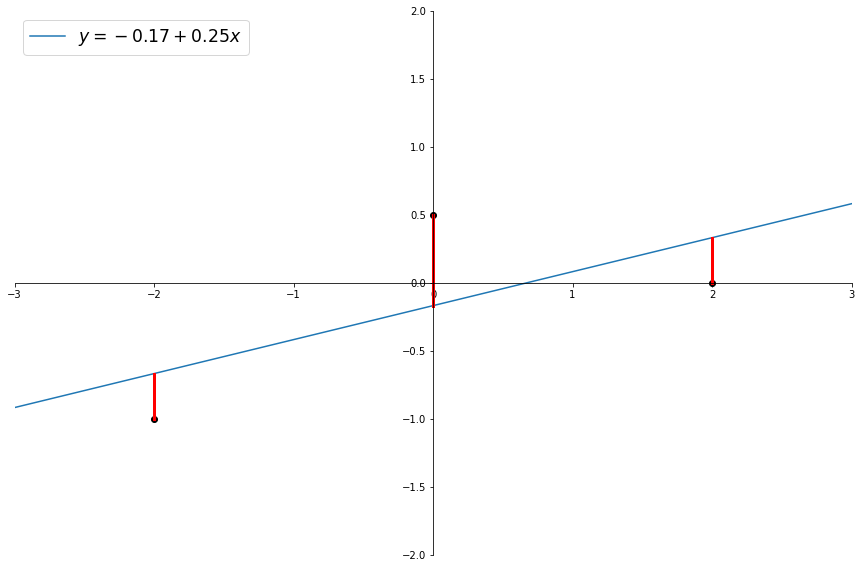# IPython Notebook Test Part 2

OddJob!!! The intercept form of the equation for a line, where the value for y is defined in terms of the value for x:

$$y = \alpha + \beta x$$

where

$\alpha$ is the intercept, where the line hits the y axis,

and

$\beta$ is the slope, how much a unit of y changes for every unit increase of x.

The following code shows a series of points that form a line and the equation corresponding to that line.

# Import the necessary libriaries
import numpy as np                                # for vectors and matrices
import matplotlib.pyplot as plt                   # for graphing

# Some housekeeping

# Output graphs in the notebook, not a separate window
%matplotlib inline
xmin = -3
xmax = 3
ymin = -2
ymax = 2

# For our purposes we'll look at 100 values of x between -5 and 5
x = np.linspace(xmin, xmax, 20)

# Define our line using the intercept and slope parameters
alpha = 1
beta = 0.5

# Calculate y for every x we have
y = alpha + beta * x

fig, ax = plt.subplots(1,1,figsize=(15.0, 10.0))

# Draw the line
ax.plot(x, y)

# Draw the points on our line
ax.scatter(x, y, color='k')

# set the x-spine (see below for more info on set_position)
ax.spines['left'].set_position('zero')

# turn off the right spine/ticks
ax.spines['right'].set_color('none')
ax.yaxis.tick_left()

# set the y-spine
ax.spines['bottom'].set_position('zero')

# turn off the top spine/ticks
ax.spines['top'].set_color('none')
ax.xaxis.tick_bottom()

ax.set_xlim(-3,3)
ax.set_ylim(-1,3)
ax.legend(labels=[f"$y = {alpha} + {beta} x$"], loc='upper left', fontsize='xx-large')

passFor a linear regression we want to draw a line with the minimal total distance to all points of interest. If we have two points we can just draw a line through them but when we have three or more points we have to discover where to draw the best line.

# Import the necessary libriaries
import numpy as np                                # for vectors and matrices
import matplotlib.pyplot as plt                   # for graphing

# Some housekeeping

# Output graphs in the notebook, not a separate window
%matplotlib inline
xmin = -3
xmax = 3
ymin = -2
ymax = 2

# First point
x1 = 0
y1 = -0.5

# Second point
x2 = 2
y2 = 0

xs = [x1, x2]
ys = [y1, y2]

beta = (y2 - y1) / (x2 - x1)
alpha = y1 - beta * x1

fig, ax = plt.subplots(1,1,figsize=(15.0, 10.0))
# Draw the line
ax.plot((xmin, xmax), (alpha + beta * xmin, alpha + beta * xmax))

# Draw the points on our line
ax.scatter(xs, ys, color='k')

# set the x-spine (see below for more info on set_position)
ax.spines['left'].set_position('zero')

# turn off the right spine/ticks
ax.spines['right'].set_color('none')
ax.yaxis.tick_left()

# set the y-spine
ax.spines['bottom'].set_position('zero')

# turn off the top spine/ticks
ax.spines['top'].set_color('none')
ax.xaxis.tick_bottom()

ax.set_xlim(xmin,xmax)
ax.set_ylim(ymin,ymax)

ax.legend(labels=[f"$y = {alpha:0.2f} + {beta:0.2f} x$"], loc='upper left', fontsize='xx-large')

passLinear Regression seeks to create a line that is as close as possible to the data points along the axis of the dependent variable (y)

# Import the necessary libriaries
import numpy as np                                # for vectors and matrices
import matplotlib.pyplot as plt                   # for graphing

# Some housekeeping

# Output graphs in the notebook, not a separate window
%matplotlib inline
xmin = -3
xmax = 3
ymin = -2
ymax = 2

# First point
x1 = -2
y1 = -1

# Second point
x2 = 0
y2 = 0.5

# Third point
x3 = 2
y3 = 0

xs = np.array([x1, x2, x3])
ys = np.array([y1, y2, y3])

n = len(xs)

# Calculate the slope and intercept of a regression line by minimizing the least squares residuals
# Closed form (using a formula) in two dimensions
beta = (n * (xs * ys).sum() - xs.sum() * ys.sum()) / (n * (xs**2).sum() - sum(xs)**2)
alpha = (ys.sum() - beta * xs.sum()) / n

fig, ax = plt.subplots(1,1,figsize=(15.0, 10.0))
# Draw the line
ax.plot((xmin, xmax), (alpha + beta * xmin, alpha + beta * xmax))
ax.legend(labels=[f"$y = {alpha:0.2f} + {beta:0.2f} x$"], loc='upper left', fontsize='xx-large')

ax.plot([x1, x1], [alpha + beta * x1, y1], c='r', lw=3)
ax.plot([x2, x2], [alpha + beta * x2, y2], c='r', lw=3)
ax.plot([x3, x3], [alpha + beta * x3, y3], c='r', lw=3)

# Draw the points
ax.scatter(xs, ys, color='k')

# set the x-spine (see below for more info on set_position)
ax.spines['left'].set_position('zero')

# turn off the right spine/ticks
ax.spines['right'].set_color('none')
ax.yaxis.tick_left()

# set the y-spine
ax.spines['bottom'].set_position('zero')

# turn off the top spine/ticks
ax.spines['top'].set_color('none')
ax.xaxis.tick_bottom()

ax.set_xlim(xmin,xmax)
ax.set_ylim(ymin,ymax)

pass